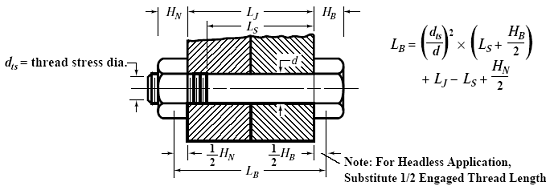Related Resources: calculators

### Bolt Elongation Equation and Calculator while under Axial Stress

Applications and Design
Stress, Deflections and Structural Analysis

Bolt Elongation Equation and Calculator while under Axial Stress

Bolt elongation is directly proportional to axial stress when the applied stress is within the elastic range of the material. If both ends of a bolt are accessible, a micrometer measurement of bolt length made before and after the application of tension will ensure the required axial stress is applied. The elongation δ in inches (mm) can be determined from the formula δ = Ft × LB ÷ E, given the required axial stress Ft in psi (MPa), the bolt modulus of elasticity E in psi (MPa), and the effective bolt length LB in inches (mm). LB, as indicated in illustration shown below, includes the contribution of bolt area and ends (head and nut) and is calculated from:Preview

Elongation

δ = Ft · LB / E

Effective length of of boltWhere:

δ = Elongation (in, mm)
Ft = Axial stress applied (psi, MPa)
LB = the effective bolt length (in, mm)
E = Modulus of Elasticity (psi, MPa)
dts = the thread stress diameter (in, mm)
d = the bolt diameter (in, mm)
Ls = the unthreaded length of the bolt shank or grip (in, mm)
Lj = the overall joint length (in, mm)
HB = the height of the bolt head (in, mm)
HN = the height of the nut (in, mm)

Related:

Reference Machinery Handbook, 23rd Edition